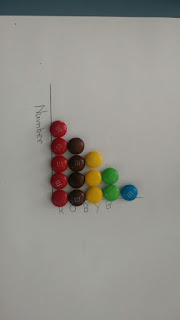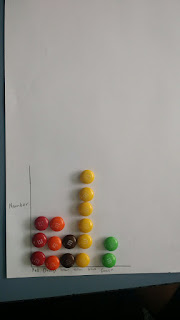Friday, 3 February 2017

Starting with Statistics

In today's maths lesson Room 8 started to discuss statistics, a topic that we will be covering every Friday. After watching a video explaining the importance of statistics, Miss Stone explained that the students would be investigating the different ways that data can be presented.

The students were asked to draw the x and y axis of a bar graph on a piece of A4 paper. A lot of the students wondered how they would be able to complete the graph as they had not collected any data. It was then that Miss Stone explained that they would be looking at the statistics of MnMs!We started by creating a strip graph.
The students created a strip, pie and bar graph using the MnMs and discussed which graph they thought shared the most information. We then began to notice how different the packs of MnMs were, as different students  received different amounts of each colour of MnMs.We then turned our strip graph into a pie graph. If we had identified the centre of the circle, we could have coloured in each segment of the pie.We found that the differences in packs became most obvious when we compared the bar charts and the pie graphs with eachother. The bar charts gave us a little more information as they told us how many MnMs in each colour we had received. Look at the difference between these bar charts!